## Example Questions

### Example Question #8 : Complex Fractions

Simplify: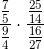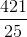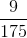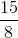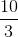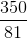Explanation:

Remember that fraction multiplication is the easiest of the arithmetical operations we can use on fractions. We can merely multiply the numerators and denominators by each other. As you will see, this is the easiest way to do this problem, for the numerators and denominators can be cancelled. Thus, we know: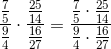Now, the parts of this fraction can be cancelled, giving us a much simpler expression: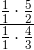, which is the same as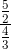To simplify this, you just need to multiply the numerator by the reciprocal of the denominator; thus, we have: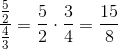### Example Question #9 : Complex Fractions

Simplify the following equation: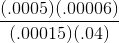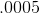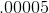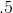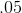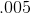Explanation:

The most important element of this question is attention to detail. It may help to rewrite the equation by cancelling out like terms in the fraction, starting with the removal of an equivalent number of zeroes from the numerator and denomerator, followed by shifting the decimals an equivalent number of spaces in the numerator and denomerator: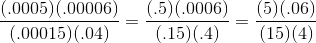Following this, like factors can be cancelled from the numerator and denominator, facilitating calculation of the answer: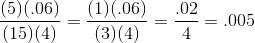### Example Question #10 : Complex Fractions

It is known thatof the athletes at a convention are volleyball players, and thatof the volley ball players are female. If there are 54 female volleyball players at the convention, how many of the athletes at the meet are not volleyball players?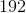Explanation:

The first step to this problem will be to find the total number of volleyball players, since the total number of athletes is related to this value. Since we know how many female volleyball players there are, we can find the total number of volleyball players by relating the proportion: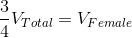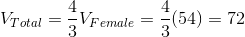This in turn allows us to find the total number of athletes: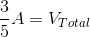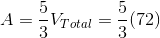And finally, from this, we can find the total number of athletes that aren't volleyball players: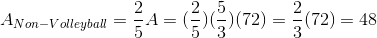Tired of practice problems?

Try live online GRE prep today.